# Disease Outbreak Response Decision-making Under Uncertainty: A retrospective analysis of measles in Sao Paulo¶

In :
%matplotlib inline
import pandas as pd
import numpy as np
import numpy.ma as ma
from datetime import datetime
import matplotlib.pyplot as plt
import seaborn as sb
sb.set()
import pdb

from pymc3 import Model, sample, find_MAP, Metropolis, NUTS
from pymc3 import (Normal, HalfCauchy, Uniform, MvNormal, Beta, Binomial,
Dirichlet, Multinomial, Bernoulli, Poisson, Wishart
)
from pymc3 import Potential, Deterministic

import theano.tensor as T

from IPython.core.display import HTML
def css_styling():
styles = open("styles/custom.css", "r").read()
return HTML(styles)
css_styling()

/usr/local/lib/python3.4/dist-packages/matplotlib/__init__.py:872: UserWarning: axes.color_cycle is deprecated and replaced with axes.prop_cycle; please use the latter.
warnings.warn(self.msg_depr % (key, alt_key))

Out:
In :
data_dir = "data/"


Import outbreak data

In :
measles_data = pd.read_csv(data_dir+"measles.csv", index_col=0)
measles_data.BIRTH = pd.to_datetime(measles_data.BIRTH)
measles_data.ONSET = pd.to_datetime(measles_data.ONSET)

In :
measles_data = (measles_data.replace({'DISTRICT': {'BRASILANDIA':'BRAZILANDIA'}})
.drop('AGE', axis=1))


Sao Paulo population by district

In :
sp_pop = pd.read_csv(data_dir+'sp_pop.csv', index_col=0)

In :
_names = sp_pop.index.values
_names[_names=='BRASILANDIA'] = 'BRAZILANDIA'
sp_pop.set_index(_names, inplace = True)

In :
sp_pop.head()

Out:
0 a 4 anos 5 a 9 anos 10 a 14 anos 15 a 19 anos 20 a 24 anos 25 a 29 anos 30 a 34 anos 35 a 39 anos 40 a 44 anos 45 a 49 anos 50 a 54 anos 55 a 59 anos 60 a 64 anos 65 a 69 anos 70 a 74 anos 75 anos e + Total
AGUA RASA 5411 5750 6450 7122 7621 7340 6999 6984 6346 5608 4987 4212 4152 3595 2937 3637 89151
ALTO DE PINHEIROS 2070 2369 2953 3661 4612 4190 3539 3633 3448 3289 3040 2533 2298 1732 1305 1823 46495
ANHANGUERA 3068 3006 2755 2431 2426 2636 2695 2308 1653 1107 753 509 352 217 162 171 26249
ARICANDUVA 7732 7730 8373 8956 9182 8531 7813 7365 6551 5554 4887 3858 3320 2449 1611 1723 95635
ARTUR ALVIM 9031 9078 10000 11058 11387 10347 9125 8658 7830 7055 5919 4612 3756 2633 1727 1724 113940

Plot of cumulative cases by district

In :
measles_onset_dist = measles_data.groupby(['DISTRICT','ONSET']).size().unstack(level=0).fillna(0)
measles_onset_dist.cumsum().plot(legend=False, grid=False)

Out:
<matplotlib.axes._subplots.AxesSubplot at 0x7fc58466c9e8>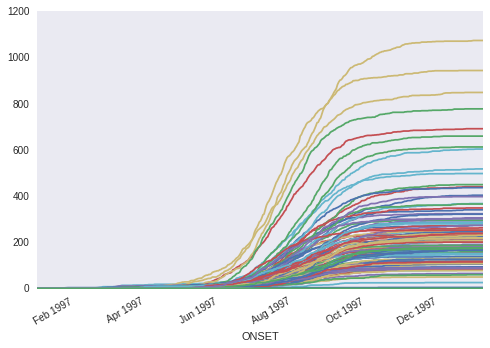In :
total_district_cases = measles_onset_dist.sum()


Top 5 districts by number of cases

In :
totals = measles_onset_dist.sum()
totals.sort(ascending=False)
totals[:5]

Out:
DISTRICT
GRAJAU             1074
JARDIM ANGELA       944
CAPAO REDONDO       849
JARDIM SAO LUIZ     778
CAMPO LIMPO         692
dtype: float64

Age distribution of cases, by confirmation status

In :
by_conclusion = measles_data.groupby(["YEAR_AGE", "CONCLUSION"])
counts_by_cause = by_conclusion.size().unstack().fillna(0)
ax = counts_by_cause.plot(kind='bar', stacked=True, xlim=(0,50), figsize=(15,5))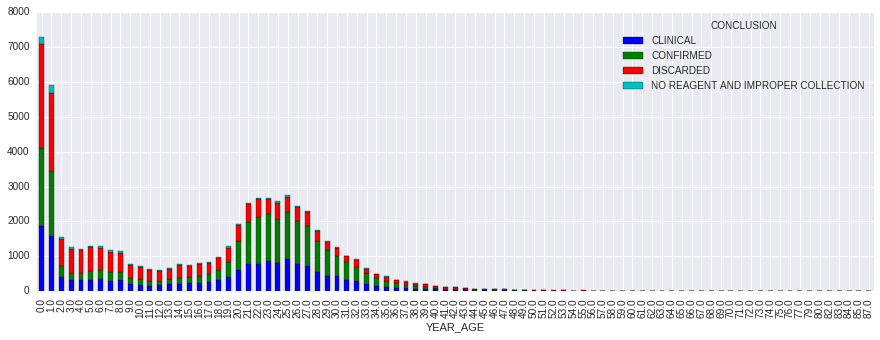### Vaccination Data¶

In :
vaccination_data = pd.read_csv('data/BrazilVaxRecords.csv', index_col=0)

Out:
BIRTHS VAX POP SIA
YEAR
1980 3896442 0.57 121740438 0
1981 3933136 0.73 124610790 0
1982 3952137 0.66 127525420 0
1983 3952735 0.68 130455659 0
1984 3935224 0.73 133364277 0
sia_susc = np.ones(len(vax_97))
birth_year = np.arange(1922, 1998)[::-1]
by_mask = (birth_year > 1983) & (birth_year < 1992)
by_mask = (birth_year > 1972) & (birth_year < 1986)

In :
vax_97 = np.r_[*(1979-1921+1), vaccination_data.VAX[:17]]
n = len(vax_97)
FOI_mat = np.resize((1 - vax_97*0.9), (n,n)).T

In :
FOI_mat

Out:
array([[ 1.   ,  1.   ,  1.   , ...,  1.   ,  1.   ,  1.   ],
[ 1.   ,  1.   ,  1.   , ...,  1.   ,  1.   ,  1.   ],
[ 1.   ,  1.   ,  1.   , ...,  1.   ,  1.   ,  1.   ],
...,
[ 0.307,  0.307,  0.307, ...,  0.307,  0.307,  0.307],
[ 0.217,  0.217,  0.217, ...,  0.217,  0.217,  0.217],
[ 0.28 ,  0.28 ,  0.28 , ...,  0.28 ,  0.28 ,  0.28 ]])
In :
vacc_susc = (1 - vax_97*0.9)[::-1]
vacc_susc = 0.5

In :
vacc_susc

Out:
array([ 0.5  ,  0.217,  0.307,  0.235,  0.181,  0.235,  0.298,  0.46 ,
0.442,  0.424,  0.397,  0.397,  0.343,  0.388,  0.406,  0.343,
0.487,  1.   ,  1.   ,  1.   ,  1.   ,  1.   ,  1.   ,  1.   ,
1.   ,  1.   ,  1.   ,  1.   ,  1.   ,  1.   ,  1.   ,  1.   ,
1.   ,  1.   ,  1.   ,  1.   ,  1.   ,  1.   ,  1.   ,  1.   ,
1.   ,  1.   ,  1.   ,  1.   ,  1.   ,  1.   ,  1.   ,  1.   ,
1.   ,  1.   ,  1.   ,  1.   ,  1.   ,  1.   ,  1.   ,  1.   ,
1.   ,  1.   ,  1.   ,  1.   ,  1.   ,  1.   ,  1.   ,  1.   ,
1.   ,  1.   ,  1.   ,  1.   ,  1.   ,  1.   ,  1.   ,  1.   ,
1.   ,  1.   ,  1.   ,  1.   ])
In :
sia_susc = np.ones(len(vax_97))
birth_year = np.arange(1922, 1998)[::-1]
by_mask = ((birth_year > 1983) & (birth_year < 1992)) | ((birth_year > 1972) & (birth_year < 1986))

In :
sia_susc

Out:
array([ 1. ,  1. ,  1. ,  1. ,  1. ,  1. ,  0.2,  0.2,  0.2,  0.2,  0.2,
0.2,  0.2,  0.2,  0.2,  0.2,  0.2,  0.2,  0.2,  0.2,  0.2,  0.2,
0.2,  0.2,  0.2,  1. ,  1. ,  1. ,  1. ,  1. ,  1. ,  1. ,  1. ,
1. ,  1. ,  1. ,  1. ,  1. ,  1. ,  1. ,  1. ,  1. ,  1. ,  1. ,
1. ,  1. ,  1. ,  1. ,  1. ,  1. ,  1. ,  1. ,  1. ,  1. ,  1. ,
1. ,  1. ,  1. ,  1. ,  1. ,  1. ,  1. ,  1. ,  1. ,  1. ,  1. ,
1. ,  1. ,  1. ,  1. ,  1. ,  1. ,  1. ,  1. ,  1. ,  1. ])

## Stochastic Disease Transmission Model¶

As a baseline for comparison, we can fit a model to all the clinically-confirmed cases, regardless of lab confirmation status. For this, we will use a simple SIR disease model, which will be fit using MCMC.

This model fits the series of 2-week infection totals in each district $i$ as a set of Poisson models:

$$Pr(I(t)_{i} | \lambda(t)_i) = \text{Poisson}(\lambda(t)_i)$$

Where the outbreak intensity is modeled as:

$$\lambda(t)_i = \beta [I^{(w)}(t-1)_i]^{\alpha} S(t-1)_i$$

$$\alpha \sim \text{Exp}(1)$$

We will assume here that the transmission rate is constant over time (and across districts):

$$\beta \sim \text{Gamma}(1, 0.1)$$

To account for the influence of infected individuals from neighboring districts on new infections, the outbreak intensity was modeled using a spatial-weighted average of infecteds across districts, where populations were weighted as an exponential function of the distance between district centroids:

$$w_{d} = \text{exp}(-\theta d)$$

$$\theta \sim \text{Exp}(1)$$

### Confirmation Sub-model¶

Rather than assume all clinical cases are true cases, we can adjust the model to account for lab confirmation probability. This is done by including a sub-model that estimates age group-specific probabilities of confirmation, and using these probabilities to estimate the number of lab-confirmed cases. These estimates are then plugged into the model in place of the clinically-confirmed cases.

We specified a structured confirmation model to retrospectively determine the age group-specific probabilities of lab confirmation for measles, conditional on clinical diagnosis. Individual lab confirmation events $c_i$ were modeled as Bernoulli random variables, with the probability of confirmation being allowed to vary by age group:

$$c_i \sim \text{Bernoulli}(p_{a(i)})$$

where $a(i)$ denotes the appropriate age group for the individual indexed by i. There were 16 age groups, the first 15 of which were 5-year age intervals $[0,5), [5, 10), \ldots , [70, 75)$, with the 16th interval including all individuals 75 years and older.

Since the age interval choices were arbitrary, and the confirmation probabilities of adjacent groups likely correlated, we modeled the correlation structure directly, using a multivariate logit-normal model. Specifically, we allowed first-order autocorrelation among the age groups, whereby the variance-covariance matrix retained a tridiagonal structure.

\begin{aligned} \Sigma = \left[{ \begin{array}{c} {\sigma^2} & {\sigma^2 \rho} & 0& \ldots & {0} & {0} \\ {\sigma^2 \rho} & {\sigma^2} & \sigma^2 \rho & \ldots & {0} & {0} \\ {0} & \sigma^2 \rho & {\sigma^2} & \ldots & {0} & {0} \\ \vdots & \vdots & \vdots & & \vdots & \vdots\\ {0} & {0} & 0 & \ldots & {\sigma^2} & \sigma^2 \rho \\ {0} & {0} & 0 & \ldots & \sigma^2 \rho & {\sigma^2} \end{array} }\right] \end{aligned}

From this, the confirmation probabilities were specified as multivariate normal on the inverse-logit scale.

$$\text{logit}(p_a) = \{a\} \sim N(\mu, \Sigma)$$

Priors for the confirmation sub-model were specified by:

\begin{aligned} \mu_i &\sim N(0, 100) \\ \sigma &\sim \text{HalfCauchy}(25) \\ \rho &\sim U(-1, 1) \end{aligned}

Age classes are defined in 5-year intervals.

In :
age_classes = [0,5,10,15,20,25,30,35,40,100]
measles_data.dropna(subset=['YEAR_AGE'], inplace=True)
measles_data['YEAR_AGE'] = measles_data.YEAR_AGE.astype(int)
measles_data['AGE_GROUP'] = pd.cut(measles_data.YEAR_AGE, age_classes, right=False)


Lab-checked observations are extracted for use in estimating lab confirmation probability.

In :
CONFIRMED = measles_data.CONCLUSION == 'CONFIRMED'
CLINICAL = measles_data.CONCLUSION == 'CLINICAL'


Extract confirmed and clinical subset, with no missing county information.

In :
lab_subset = measles_data[(CONFIRMED | CLINICAL) & measles_data.COUNTY.notnull()].copy()

In :
age = lab_subset.YEAR_AGE.values
ages = lab_subset.YEAR_AGE.unique()
counties = lab_subset.COUNTY.unique()
confirmed = (lab_subset.CONCLUSION=='CONFIRMED').values

In :
clinic_subset = measles_data[CLINICAL & measles_data.COUNTY.notnull()].copy()

In :
_lab_subset = lab_subset.replace({"CONCLUSION": {"CLINICAL": "UNCONFIRMED"}})
by_conclusion = _lab_subset.groupby(["YEAR_AGE", "CONCLUSION"])
counts_by_cause = by_conclusion.size().unstack().fillna(0)
ax = counts_by_cause.plot(kind='bar', stacked=True, xlim=(0,50),
figsize=(15,5), grid=False)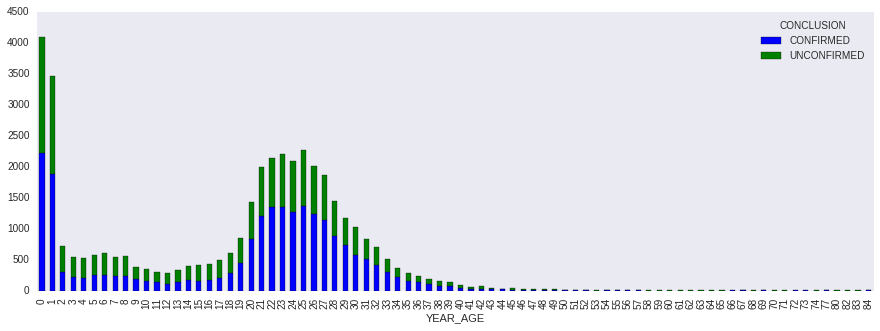In :
lab_subset.shape

Out:
(39982, 15)
In :
confirmed.sum()

Out:
22097

Proportion of lab-confirmed cases older than 20 years

In :
(measles_data[CONFIRMED].YEAR_AGE>20).mean()

Out:
0.60257048468117846
In :
age_classes

Out:
[0, 5, 10, 15, 20, 25, 30, 35, 40, 100]
In :
age_group = pd.cut(age, age_classes, right=False)
age_index = np.array([age_group.categories.tolist().index(i) for i in age_group])
age_groups = age_group.categories
age_groups

Out:
Index(['[0, 5)', '[5, 10)', '[10, 15)', '[15, 20)', '[20, 25)', '[25, 30)',
'[30, 35)', '[35, 40)', '[40, 100)'],
dtype='object')
In :
#Extract cases by age and time.
age_group = pd.cut(age, age_classes, right=False)
age_index = np.array([age_group.categories.tolist().index(i) for i in age_group])

In :
age_group.categories

Out:
Index(['[0, 5)', '[5, 10)', '[10, 15)', '[15, 20)', '[20, 25)', '[25, 30)',
'[30, 35)', '[35, 40)', '[40, 100)'],
dtype='object')
In :
age_slice_endpoints = [g[1:-1].split(',') for g in age_groups]
age_slices = [slice(int(i), int(i)) for i in age_slice_endpoints]

In :
# Get index from full crosstabulation to use as index for each district
dates_index = measles_data.groupby(
['ONSET', 'AGE_GROUP']).size().unstack().index

In :
unique_districts = measles_data.DISTRICT.dropna().unique()

In :
excludes = ['BOM RETIRO']

In :
N = sp_pop.drop(excludes).ix[unique_districts].sum().drop('Total')

In :
N_age = N.iloc[:8]
N_age.index = age_groups[:-1]
N_age[age_groups[-1]] = N.iloc[8:].sum()
N_age

Out:
[0, 5)        844130
[5, 10)       830880
[10, 15)      858750
[15, 20)      904972
[20, 25)      945244
[25, 30)      902086
[30, 35)      835888
[35, 40)      764605
[40, 100)    2841133
dtype: float64

Compile bi-weekly confirmed and unconfirmed data by Sao Paulo district

In :
# All confirmed cases, by district
confirmed_data = lab_subset[lab_subset.CONCLUSION=='CONFIRMED']
confirmed_counts = (confirmed_data.groupby(['ONSET', 'AGE_GROUP'])
.size()
.unstack()
.reindex(dates_index)
.fillna(0)
.sum())

all_confirmed_cases = (confirmed_counts.reindex_axis(measles_data['AGE_GROUP'].unique())
.fillna(0).values.astype(int))

In :
confirmed_counts_2w = (confirmed_data
.groupby(['ONSET', 'AGE_GROUP'])
.size()
.unstack()
.reindex(dates_index)
.fillna(0)
.resample('2W', how='sum'))

In :
# All clinical cases, by district
clinical_counts = (clinic_subset.groupby(['ONSET', 'AGE_GROUP'])
.size()
.unstack()
.reindex(dates_index)
.fillna(0)
.sum())

all_clinical_cases = (clinical_counts.reindex_axis(measles_data['AGE_GROUP'].unique())
.fillna(0).values.astype(int))

In :
clinical_counts_2w = (clinic_subset
.groupby(['ONSET', 'AGE_GROUP'])
.size()
.unstack()
.reindex(dates_index)
.fillna(0)
.resample('2W', how='sum'))

In :
age_groups = np.sort(measles_data['AGE_GROUP'].unique())
age_groups

Out:
array(['[0, 5)', '[10, 15)', '[15, 20)', '[20, 25)', '[25, 30)',
'[30, 35)', '[35, 40)', '[40, 100)', '[5, 10)'], dtype=object)

Check shape of data frame

• 28 bi-monthly intervals, 9 age groups
In :
assert clinical_counts_2w.shape == (28, len(age_groups))

In :
confirmed_counts_2w

Out:
AGE_GROUP [0, 5) [5, 10) [10, 15) [15, 20) [20, 25) [25, 30) [30, 35) [35, 40) [40, 100)
ONSET
1997-01-05 0 0 0 0 1 0 0 0 0
1997-01-19 0 1 0 0 3 4 0 0 0
1997-02-02 4 1 0 0 2 1 0 0 0
1997-02-16 4 0 0 0 2 1 1 0 0
1997-03-02 9 0 0 2 4 5 1 0 1
1997-03-16 13 2 1 3 12 17 4 0 0
1997-03-30 17 2 2 3 6 5 1 0 0
1997-04-13 18 3 0 1 7 10 3 1 0
1997-04-27 17 3 4 5 23 10 4 0 1
1997-05-11 31 4 5 8 32 37 11 5 0
1997-05-25 59 10 7 11 71 49 12 3 4
1997-06-08 91 11 18 18 109 105 31 10 3
1997-06-22 212 28 18 38 250 209 65 17 10
1997-07-06 380 62 35 92 547 435 155 46 17
1997-07-20 567 104 46 146 765 652 252 61 32
1997-08-03 815 146 119 178 904 728 250 70 25
1997-08-17 835 167 107 167 753 737 262 71 25
1997-08-31 571 200 89 169 742 716 257 77 37
1997-09-14 417 150 103 171 690 616 252 79 25
1997-09-28 219 111 51 104 421 348 159 51 29
1997-10-12 151 50 31 66 231 247 103 27 9
1997-10-26 97 21 20 25 103 113 61 16 3
1997-11-09 71 32 14 10 84 64 34 11 5
1997-11-23 49 20 2 4 40 42 25 1 2
1997-12-07 16 3 3 7 26 33 9 5 0
1997-12-21 23 3 1 4 9 22 4 1 1
1998-01-04 7 0 0 1 5 3 3 1 2
1998-01-18 0 0 0 0 1 2 0 0 0
In :
clinical_counts_2w

Out:
AGE_GROUP [0, 5) [5, 10) [10, 15) [15, 20) [20, 25) [25, 30) [30, 35) [35, 40) [40, 100)
ONSET
1997-01-05 3 1 1 0 0 0 0 0 0
1997-01-19 30 3 1 1 1 3 2 1 0
1997-02-02 22 4 0 2 1 1 1 0 1
1997-02-16 21 2 2 2 2 1 1 0 2
1997-03-02 24 5 2 5 2 2 2 1 0
1997-03-16 39 11 4 10 7 2 3 0 2
1997-03-30 33 5 2 3 3 1 0 1 1
1997-04-13 24 3 1 4 6 3 1 0 2
1997-04-27 31 5 2 4 5 6 2 2 0
1997-05-11 36 8 4 5 10 9 6 1 2
1997-05-25 40 15 5 7 22 23 6 1 2
1997-06-08 57 15 9 13 48 48 16 2 2
1997-06-22 126 26 14 37 107 105 30 9 8
1997-07-06 239 35 44 66 254 183 79 19 17
1997-07-20 359 92 51 108 403 380 147 26 31
1997-08-03 475 125 68 140 534 463 179 67 23
1997-08-17 639 179 116 197 593 514 214 45 43
1997-08-31 581 214 155 278 568 537 217 83 54
1997-09-14 465 248 161 252 522 445 206 67 50
1997-09-28 315 165 135 160 289 281 103 29 38
1997-10-12 213 92 39 56 135 135 60 20 17
1997-10-26 142 52 28 18 77 77 36 11 7
1997-11-09 112 45 15 17 38 24 9 3 5
1997-11-23 78 21 9 10 32 20 11 5 0
1997-12-07 74 12 4 13 6 6 6 2 2
1997-12-21 57 10 8 4 11 7 5 1 1
1998-01-04 31 5 2 2 2 2 1 0 1
1998-01-18 2 0 0 0 0 0 1 0 0
In :
obs_date = '1997-06-15'
confirmed_obs_t = confirmed_counts_2w[confirmed_counts_2w.index <= obs_date].values
clinical_obs_t = clinical_counts_2w[clinical_counts_2w.index <= obs_date].values

In :
model_june = Model()
model_july = Model()
model_june_noconf = Model()
model_july_noconf = Model()

In :
N_age.values.astype(int)

Out:
array([ 844130,  830880,  858750,  904972,  945244,  902086,  835888,
764605, 2841133])
In :
# Downsample annual series to observed age groups
downsample = lambda x: np.array([x[s].mean() for s in age_slices])

In :
invlogit = T.nnet.sigmoid

confirmation = True

def specify_model(model, observation_date, confirmation=True):

with model:

n_periods, n_age_groups = confirmed_counts_2w.shape

### Confirmation sub-model

if confirmation:

# Specify priors on age-specific means
age_classes = np.unique(age_index)

p_age = Beta('p_age', 1, 1, shape=n_age_groups)
p_confirm = p_age[age_index]

# Confirmation likelihood
lab_confirmed = Bernoulli('lab_confirmed', p=p_confirm,
observed=confirmed)

'''
Truncate data at observation period
'''
obs_index = confirmed_counts_2w.index <= observation_date
confirmed_obs_t = confirmed_counts_2w[obs_index].values
clinical_obs_t = clinical_counts_2w[obs_index].values

# Index for observation date, used to index out values of interest
# from the model.
t_obs = obs_index.sum() - 1

if confirmation:

clinical_cases = [Binomial('clinical_cases_%i' % n, i,
p_age,
shape=n_age_groups) for n,i in enumerate(clinical_obs_t)]
I_age = [Deterministic('I_age_%i' % i, clinical + confirmed_obs_t[i])
for i,clinical in enumerate(clinical_cases)]
I = T.stacklists(I_age)

else:

I = confirmed_obs_t + clinical_obs_t

# Calcuate age distribution from observed distribution of infecteds to date
age_dist = Dirichlet('age_dist', np.ones(n_age_groups),
shape=n_age_groups)

age_dist_like = Potential('age_dist_like',
Multinomial.dist(I.sum(), age_dist).logp(I.sum(0)))

# Transmission parameter
beta = Uniform('beta', 1, 100)

A = 75./(beta - 1)
lt_sum = downsample(np.tril(FOI_mat).sum(0)[::-1])
natural_susc = Deterministic('natural_susc', T.exp((-1/A) * lt_sum))

# Weakly-informative prior on proportion susceptible being
# between 0 and 0.07
#         natural_susc = Beta('natural_susc', 2, 100, shape=n_age_groups)
p_susceptible = Deterministic('p_susceptible',
downsample(sia_susc) * downsample(vacc_susc) * natural_susc)

# Estimated total initial susceptibles
S_0 = Binomial('S_0', n=N_age.values.astype(int), p=p_susceptible,
shape=n_age_groups)

S = Deterministic('S', S_0 - I.cumsum(axis=0))

# Force of infection
λ = Deterministic('λ', beta * I.sum(1) * S.sum(1) / N_age.sum())

new_cases = Potential('new_cases', Poisson.dist(λ[:-1]).logp(I.sum(1)[1:]))

return model

In :
from theano import config
config.warn.reduce_join = False


Run models for June 15 and July 15 observation points, both with and without clinical confirmation.

In [ ]:
n_iterations = 100000
n_burn = 90000


June 15, with lab confirmation

In [ ]:
model_june = specify_model(model_june, '1997-06-15')

with model_june:
june_trace = sample(n_iterations)

Applied logodds-transform to p_age and added transformed p_age_logodds to model.
Applied stickbreaking-transform to age_dist and added transformed age_dist_stickbreaking to model.
Applied interval-transform to beta and added transformed beta_interval to model.
Assigned NUTS to p_age_logodds

July 15, with lab confirmation

In [ ]:
model_july = specify_model(model_july, '1997-07-15')

with model_july:
july_trace = sample(n_iterations)


June 15, no lab confirmation

In [ ]:
model_june_noconf = specify_model(model_june_noconf, '1997-06-15',
confirmation=False)

with model_june_noconf:
june_noconf_trace = sample(n_iterations)


July 15, no lab confirmation

In [ ]:
model_july_noconf = specify_model(model_july_noconf, '1997-07-15',
confirmation=False)

with model_july_noconf:
july_noconf_trace = sample(n_iterations)


## Summary of model output¶

Age-specific confirmation, June model

In :
from pymc3 import traceplot, forestplot

forestplot(june_trace[n_burn:], vars=['p_age'])

Out:
<matplotlib.gridspec.GridSpec at 0x7fc520dbc400>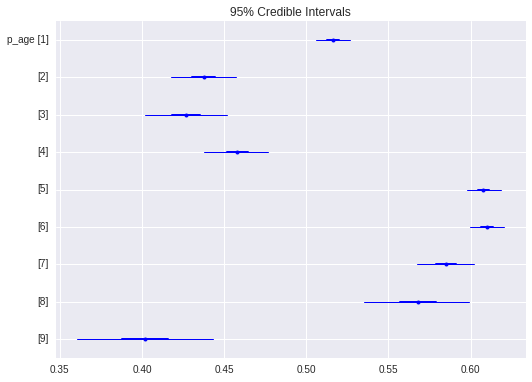Lab confirmation rates, June model

In :
p_age = pd.DataFrame(june_trace.get_values('p_age'), columns=age_groups)

f, axes = plt.subplots(figsize=(14,6))
sb.boxplot(data=p_age, linewidth=0.3, fliersize=0, ax=axes,
color=sb.color_palette("coolwarm", 5),
order=age_group.categories)
axes.set_ylabel('Confirmation rate')
axes.set_xlabel('Age group')

Out:
<matplotlib.text.Text at 0x7fc51f5cc780>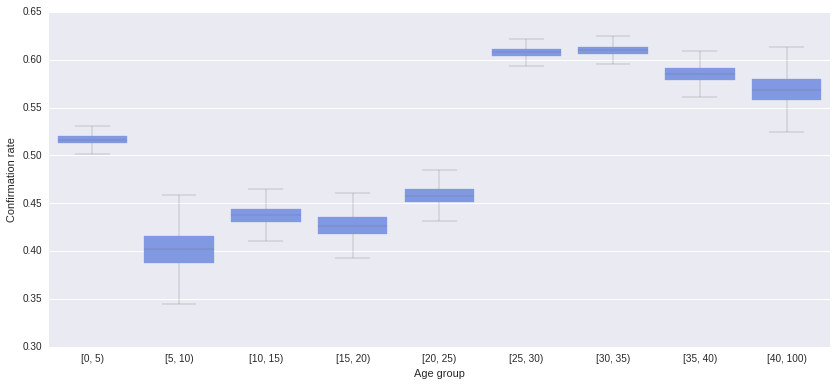Proportion of population susceptible, June model.

In :
forestplot(june_trace, vars=['p_susceptible'])

Out:
<matplotlib.gridspec.GridSpec at 0x7fc520427f60>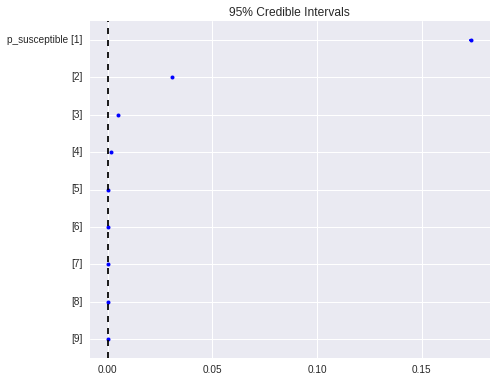Proportion of population susceptible, June model with no confirmation correction

In :
forestplot(june_noconf_trace[n_burn:], vars=['p_susceptible'])

Out:
<matplotlib.gridspec.GridSpec at 0x7fc51d12c2e8>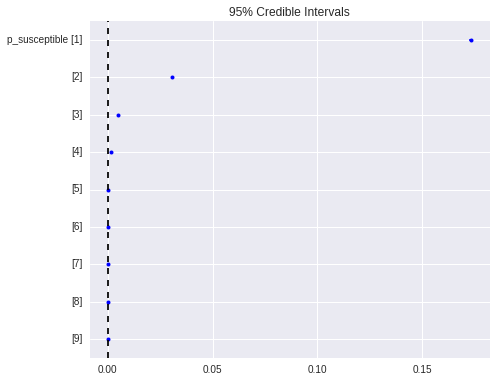Epidemic intensity estimates at June and July.

In :
forestplot(june_trace[n_burn:], vars=['λ'])

Out:
<matplotlib.gridspec.GridSpec at 0x7fc51c7aa748>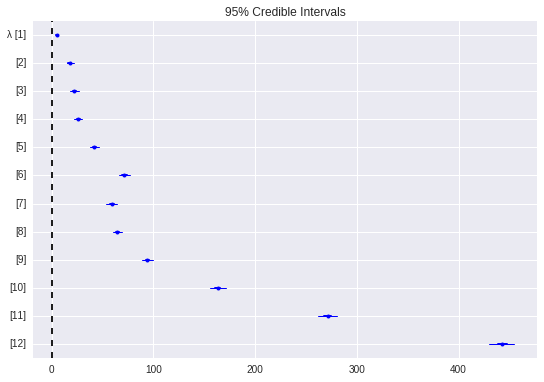In :
forestplot(july_trace[n_burn:], vars=['λ'])

Out:
<matplotlib.gridspec.GridSpec at 0x7fc53c6345f8>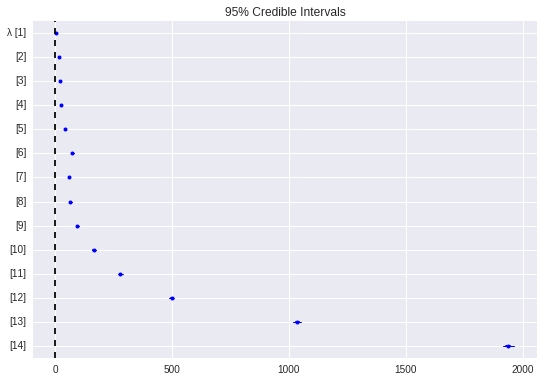Transmission paramter

In :
traceplot(june_trace[n_burn:], vars=['beta'])

Out:
array([[<matplotlib.axes._subplots.AxesSubplot object at 0x7fc51f8387f0>,
<matplotlib.axes._subplots.AxesSubplot object at 0x7fc51cf9d390>]], dtype=object)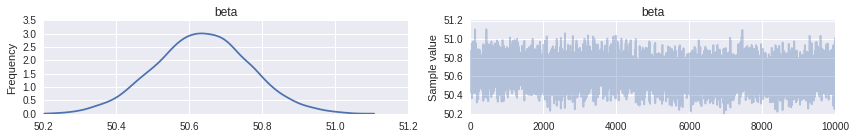In :
traceplot(june_noconf_trace[n_burn:], vars=['beta'])

Out:
array([[<matplotlib.axes._subplots.AxesSubplot object at 0x7fc522143400>,
<matplotlib.axes._subplots.AxesSubplot object at 0x7fc51d09fd30>]], dtype=object)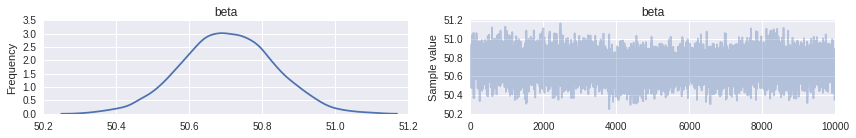Epidemic intensity for lab- versus clinical-confirmation models

In :
from pymc3 import df_summary

In :
lam_june = df_summary(june_trace[n_burn:], vars='λ')

fig, axes = plt.subplots(2, 1, sharey=True)

lam_june['mean'].plot(ax=axes, alpha=0.4)
axes.set_ylabel('Epidemic intensity')
axes.set_xlabel('time (2-week periods)')
axes.set_title('Lab confirmation')

lam_june_noconf = df_summary(june_noconf_trace[n_burn:], vars='λ')

lam_june_noconf['mean'].plot(ax=axes, alpha=0.4)
axes.set_ylabel('Epidemic intensity')
axes.set_xlabel('time (2-week periods)')
axes.set_title('Clinical confirmation')

plt.tight_layout()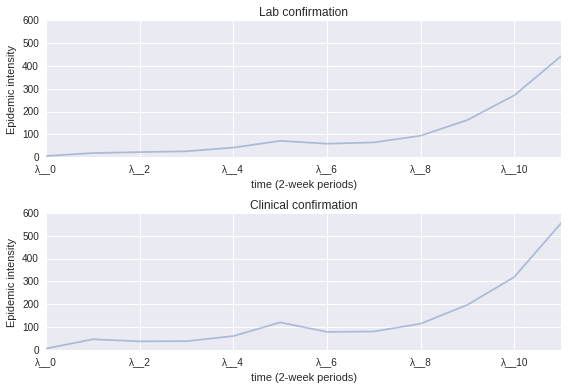In :
plt.boxplot(july_trace.get_values('S')[n_burn:,-1,:]);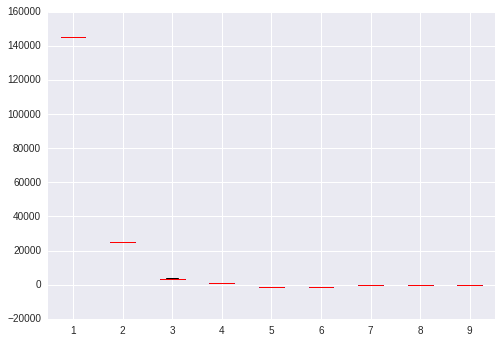In :
S_age_june = pd.DataFrame(june_trace.get_values('S')[n_burn:, -1, :],
columns=age_group.categories).unstack().reset_index()
S_age_june.columns = 'Age', 'Iteration', 'S'
S_age_june['Confirmation'] = 'Lab'

In :
S_age_june = pd.DataFrame(june_trace.get_values('S')[n_burn:, -1, :],
columns=age_group.categories).unstack().reset_index()
S_age_june.columns = 'Age', 'Iteration', 'S'
S_age_june['Confirmation'] = 'Lab'

S_age_june_noconf = pd.DataFrame(june_noconf_trace.get_values('S')[n_burn:, -1, :],
columns=age_group.categories).unstack().reset_index()
S_age_june_noconf.columns = 'Age', 'Iteration', 'S'
S_age_june_noconf['Confirmation'] = 'Clinical'

S_age_june = pd.concat([S_age_june, S_age_june_noconf], ignore_index=True)

In :
S_age_july = pd.DataFrame(july_trace.get_values('S')[n_burn:, -1, :],
columns=age_group.categories).unstack().reset_index()
S_age_july.columns = 'Age', 'Iteration', 'S'
S_age_july['Confirmation'] = 'Lab'

S_age_july_noconf = pd.DataFrame(june_noconf_trace.get_values('S')[n_burn:, -1, :],
columns=age_group.categories).unstack().reset_index()
S_age_july_noconf.columns = 'Age', 'Iteration', 'S'
S_age_july_noconf['Confirmation'] = 'Clinical'

S_age_july = pd.concat([S_age_july, S_age_july_noconf], ignore_index=True)


Numbers of suscepibles in each age group, under lab vs clinical confirmation

In :
g = sb.factorplot("Age", "S", "Confirmation", S_age_june, kind="box",
palette="hls", size=6, aspect=2, linewidth=0.3, fliersize=0,
order=age_group.categories)
g.despine(offset=10, trim=True)
g.set_axis_labels("Age Group", "Susceptibles");

/usr/local/lib/python3.4/dist-packages/matplotlib/__init__.py:892: UserWarning: axes.color_cycle is deprecated and replaced with axes.prop_cycle; please use the latter.
warnings.warn(self.msg_depr % (key, alt_key))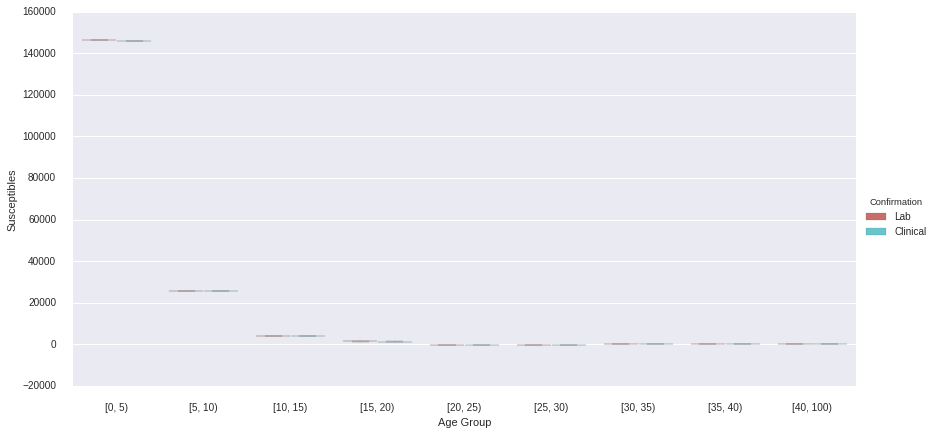In :
g = sb.factorplot("Age", "S", "Confirmation", S_age_july, kind="box",
palette="hls", size=6, aspect=2, linewidth=0.3, fliersize=0,
order=age_group.categories)
g.despine(offset=10, trim=True)
g.set_axis_labels("Age Group", "Susceptibles");

/usr/local/lib/python3.4/dist-packages/matplotlib/__init__.py:892: UserWarning: axes.color_cycle is deprecated and replaced with axes.prop_cycle; please use the latter.
warnings.warn(self.msg_depr % (key, alt_key))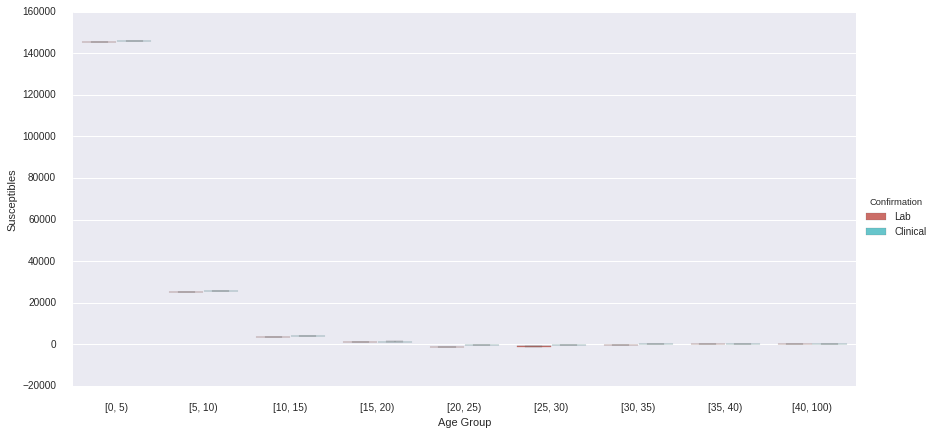## Vaccination coverage¶

In :
n_age_groups = len(age_groups)

coverage_5 = np.r_[[0.95], *(n_age_groups - 1)]
coverage_15 = np.r_[[0.95]*3, *(n_age_groups - 3)]
coverage_30 = np.r_[[0.95]*6, *(n_age_groups - 6)]
coverage_adult = np.r_[[0.95, 0, 0, 0, 0.95, 0.95] + *(n_age_groups - 6)]

coverage_dict = {'pct_5': coverage_5,
'pct_15': coverage_15,
'pct_30': coverage_30,

In :
def calc_coverage(trace, p):
n = trace.get_values('S')[:,-1,:].copy().astype(int)
n[n<0] = 0
vacc = np.random.binomial(n, p).sum(1)
pct = vacc/trace.get_values('S')[:,-1,:].sum(1)

return pct

In :
labels = ['pct_5', 'pct_15', 'pct_30', 'pct_adult']

june_coverage = pd.DataFrame({v: calc_coverage(june_trace[n_burn:],
coverage_dict[v]) for v in labels})
june_coverage['Month'] = 'June'
june_coverage['Confirmation'] = 'Lab'

In :
june_noconf_coverage = pd.DataFrame({v: calc_coverage(june_noconf_trace[n_burn:],
coverage_dict[v]) for v in labels})
june_noconf_coverage['Month'] = 'June'
june_noconf_coverage['Confirmation'] = 'Clinical'

july_coverage = pd.DataFrame({v: calc_coverage(july_trace[n_burn:],
coverage_dict[v]) for v in labels})
july_coverage['Month'] = 'July'
july_coverage['Confirmation'] = 'Lab'

july_noconf_coverage = pd.DataFrame({v: calc_coverage(july_noconf_trace[n_burn:],
coverage_dict[v]) for v in labels})
july_noconf_coverage['Month'] = 'July'
july_noconf_coverage['Confirmation'] = 'Clinical'

In :
coverage = pd.concat([june_coverage, june_noconf_coverage, july_coverage, july_noconf_coverage],
ignore_index=True)

In :
sb.factorplot(row="Month", col="Confirmation", data=coverage, kind='box',
row_order=['June', 'July'],
order=['pct_5', 'pct_15', 'pct_30', 'pct_adult'],
palette="YlGnBu_d", linewidth=0.7, fliersize=0, aspect=1.25).despine(left=True)

/usr/local/lib/python3.4/dist-packages/matplotlib/__init__.py:892: UserWarning: axes.color_cycle is deprecated and replaced with axes.prop_cycle; please use the latter.
warnings.warn(self.msg_depr % (key, alt_key))

Out:
<seaborn.axisgrid.FacetGrid at 0x7fc520d09e10>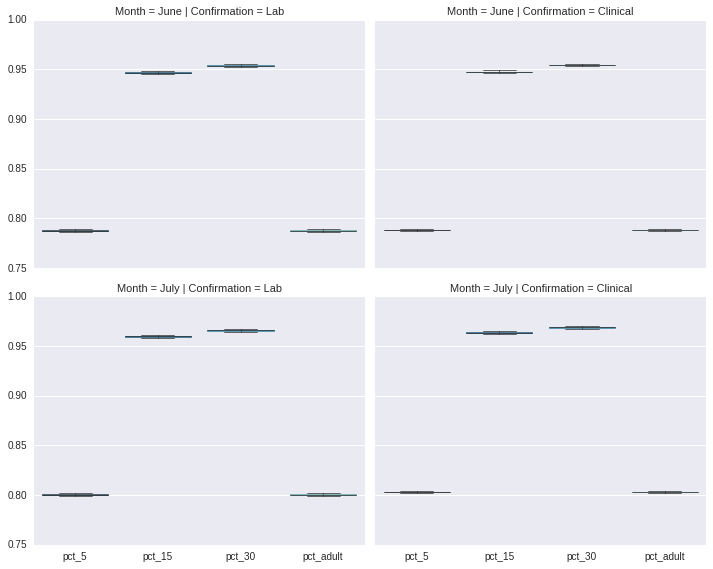## Mapping spatial effects¶

In [ ]:
from mpl_toolkits.basemap import Basemap
import geopandas as gpd

lllat=-24
urlat=-23.3
lllon=-47
urlon=-46.3

SP_base = Basemap(ax=None, lon_0=(urlon + lllon) / 2, lat_0=(urlat + lllat) / 2,
llcrnrlat=lllat, urcrnrlat=urlat, llcrnrlon=lllon, urcrnrlon=urlon,
resolution='i',
epsg='4326')

In [ ]:
SP_dist = gpd.GeoDataFrame.from_file('Sao Paulo/Brazil_full/BRA_adm3.shp').to_crs({'proj': 'longlat',
'ellps': 'WGS84',
'datum': 'WGS84'})

In [ ]:
SP_dist['DIST_NAME'] = [trans.trans(_).upper() for _ in SP_dist.NAME_3]

In [ ]:
λ_june = pd.Series(model_june.λ_t.stats()['mean'], index=sp_districts)

In [ ]:
λ_june

In [ ]:
SP_dist_merged = SP_dist.merge(pd.DataFrame(λ_june, columns=['λ']), left_on='DIST_NAME', right_index=True)

In [ ]:
measles_onset_conf = measles_data[CONFIRMED].groupby(['DISTRICT','ONSET']).size().unstack(level=0).fillna(0).sum()

In [ ]:
measles_onset_conf

In [ ]:
_rates = measles_onset_conf/sp_pop.sum(1)

In [ ]:
SP_dist_conf = SP_dist.merge(pd.DataFrame(_rates, columns=['rate']), left_on='DIST_NAME', right_index=True)


Estimated expected value for infecteds, by district

In [ ]:
from matplotlib.pyplot import cm

map_fig = plt.figure(figsize=(16,12))
map_ax = plt.gca()
SP_base.drawcoastlines()
SP_base.drawrivers()

SP_dist_merged.plot(column='λ', colormap=cm.Reds, axes=map_ax)


Observed confirmed cases, by district

In [ ]:
map_fig = plt.figure(figsize=(16,12))
map_ax = plt.gca()
SP_base.drawcoastlines()
SP_base.drawrivers()

SP_dist_conf.plot(column='rate', colormap=cm.Reds, axes=map_ax)